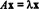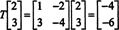## Eigenvalue and Eigenvector Defined

Although the process of applying a linear operator T to a vector gives a vector in the same space as the original, the resulting vector usually points in a completely different direction from the original, that is, T( x) is neither parallel nor antiparallel to x. However, it can happen that T( x) is a scalar multiple of x—even when x ≠ 0—and this phenomenon is so important that it deserves to be explored.

If T : R n R n is a linear operator, then T must be given by T( x) = A x for some n x n matrix A. If x ≠ 0 and T( x) = A x is a scalar multiple of x, that is, iffor some scalar λ, then λ is said to be an eigenvalue of T (or, equivalently, of A). Any nonzero vector x which satisfies this equation is said to be an eigenvector of T (or of A) corresponding to λ. To illustrate these definitions, consider the linear operator T : R 2R 2 defined by the equationThat is, T is given by left multiplication by the matrixConsider, for example, the image of the vector x = (1, 3) T under the action of T:Clearly, T( x) is not a scalar multiple of x, and this is what typically occurs.

However, now consider the image of the vector x = (2, 3) T under the action of T:Here, T( x) is a scalar multiple of x, since T( x) = (−4, −6) T = −2(2, 3) T = −2 x. Therefore, −2 is an eigenvalue of T, and (2, 3) T is an eigenvector corresponding to this eigenvalue. The question now is, how do you determine the eigenvalues and associated eigenvectors of a linear operator?